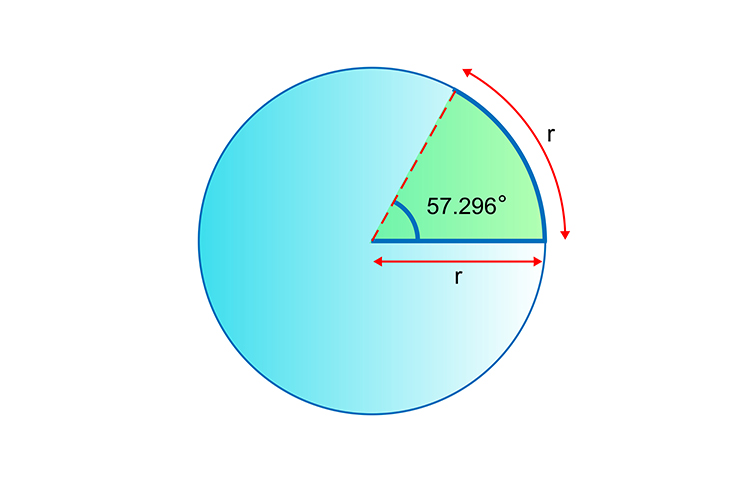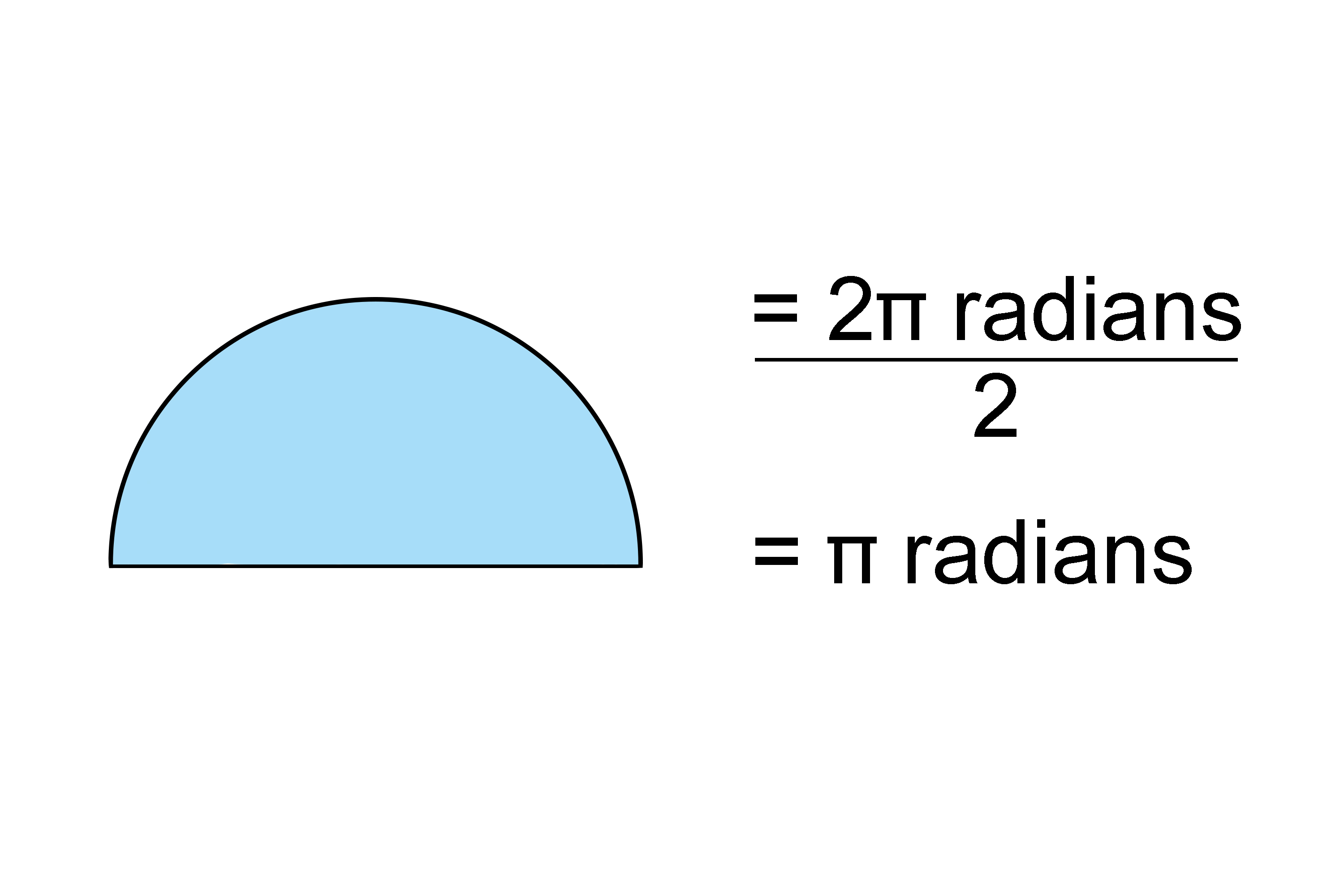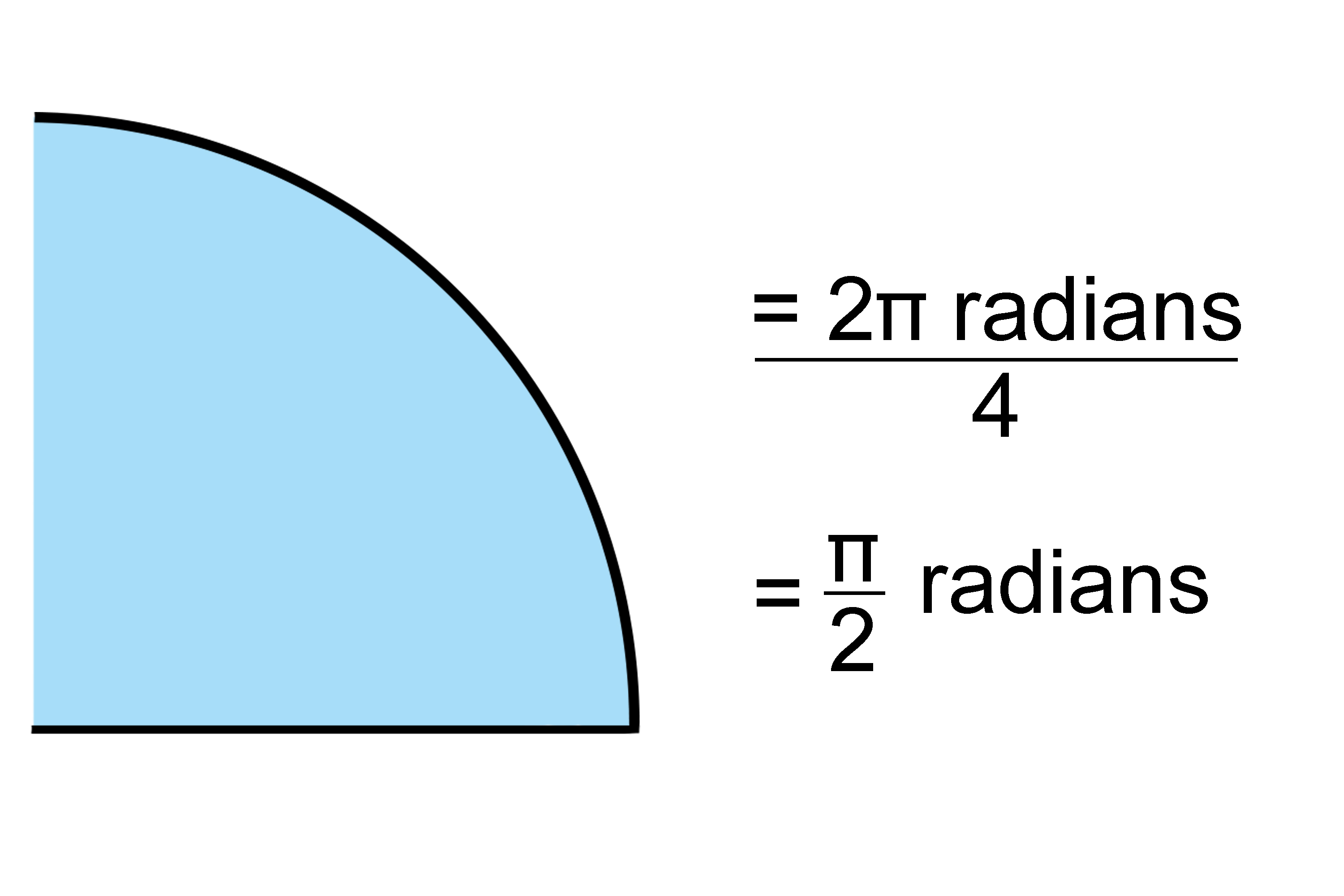# How many radians in a full circle?

How many radians are there in a full circle can be re-written as:

How many 57.296^@ are there in a full circle?

### To work this out

360^@/57.296^@=6.283

Answer:  =6.283

## Another way that this can be expressed isCircumference=2pir

Circumference=2pi radians (radius lengths)

Circumference=2pi radians

NOTE:

1.  The arc of a semi-circle would be ½ of this2.  A quarter of the arc of a circle would be a ¼ of 2pi radiansIn fact

Any part of a circle can be expressed in terms of radians (or parts of 57.269^@)